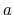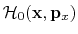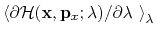Next: Implementation of the free Up: Alchemical Free Energy Methods1 Previous: Alchemical Free Energy Methods1   Contents   Index

Subsections

Theoretical Background

Free energy differences can be obtained through four different routes: (i) probability densities, (ii) free energy perturbation, (iii) thermodynamic integration, or (iv) nonequilibrium work approaches . Within NAMD, alchemical transformations are modeled following the second and the third routes, both of which rely upon the use of a general extent parameter often referred to as the coupling parameter [8,71,54,55] for the description of chemical changes in the molecular systems between the reference and the target states.

In a typical alchemical transformation setup involving the alteration of one chemical species into an alternate one in the course of the simulation, the atoms in the molecular topology can be classified into three groups, (i) a group of atoms that do not change during the simulation -- e.g. the environment, (ii) the atoms describing the reference state,, of the system, and (iii) the atoms that correspond to the target state,, at the end of the alchemical transformation. The atoms representative of stateshould never interact with those of statethroughout the MD simulation. Such a setup, in which atoms of both the initial and the final states of the system are present in the molecular topology file -- i.e. the psf file -- is characteristic of the so-called dual topology'' paradigm [37,86,3]. The hybrid Hamiltonian of the system is a function of the general extent parameter,, which connects smoothly stateto state. In the simplest case, such a connection may be achieved by linear combination of the corresponding Hamiltonians:(76)

wheredescribes the interaction of the group of atoms representative of the reference state,, with the rest of the system.characterizes the interaction of the target topology,, with the rest of the system.is the Hamiltonian describing those atoms that do not undergo any transformation during the MD simulation.

For instance, in the point mutation of an alanine side chain into that of glycine, by means of a free energy calculation -- either free energy perturbation or thermodynamic integration, the topology of both the methyl group of alanine and the hydrogen borne by the Cin glycine co-exist throughout the simulation (see Figure 6), yet without actually seeing each other.The energy and forces are defined as a function of, in such a fashion that the interaction of the methyl group of alanine with the rest of the protein is effective at the beginning of the simulation, i.e.= 0, while the glycine Chydrogen atom does not interact with the rest of the protein, and vice versa at the end of the simulation, i.e.= 1. For intermediate values of, both the alanine and the glycine side chains participate in nonbonded interactions with the rest of the protein, scaled on the basis of the current value of. It should be clearly understood that these side chains never interact with each other.

It is noteworthy that end points of alchemical transformations carried out in the framework of the dual-topology paradigm have been shown to be conducive to numerical instabilities from molecular dynamics simulations, often coined as end-point catastrophes''. These scenarios are prone to occur whenbecomes close to 0 or 1, and incoming atoms instantly appear where other particles are already present, which results in a virtually infinite potential as the interatomic distance tends towards 0. Such end-point catastrophes'' can be profitably circumvented by introducing a so-called soft-core potential [7,67], aimed at a gradual scaling of the short-range nonbonded interactions of incoming atoms with their environment, as shown in Equation 78. What is really being modified is the value of a coupling parameter (or) that scales the interactions -- i.e., if set to 0, the latter are off; if set to 1, they are on -- in lieu of the actual value ofprovided by the user.(77)

It is also worth noting that the free energy calculation does not alter intermolecular bonded potentials, e.g. bond stretch, valence angle deformation and torsions, in the course of the simulation. In calculations targeted at the estimation of free energy differences between two states characterized by distinct environments -- e.g. a ligand, bound to a protein in the first simulation, and solvated in water, in the second -- as is the case for most free energy calculations that make use of a thermodynamic cycle, perturbation of intramolecular terms may, by and large, be safely avoided . This property is controlled by the alchDecouple keyword described in

Free Energy Perturbation

Within the FEP framework  [8,21,22,38,57,71,109,112,122], the free energy difference between two alternate states,and, is expressed by:(78)

Here,, whereis the Boltzmann constant,is the temperature.andare the Hamiltonians describing statesand, respectively.denotes an ensemble average over configurations representative of the initial, reference state,.(a)(b)(c)

Convergence of equation (79) implies that low-energy configurations of the target state,, are also configurations of the reference state,, thus resulting in an appropriate overlap of the corresponding ensembles -- see Figure 7. Transformation between the two thermodynamic states is replaced by a series of transformations between non-physical, intermediate states along a well-delineated pathway that connectsto. This pathway is characterized by the general extent parameter [8,54,55,71],, that makes the Hamiltonian and, hence, the free energy, a continuous function of this parameter betweenand:(79)

Here,stands for the number of intermediate stages, or windows'' between the initial and the final states -- see Figure 7.

Thermodynamic Integration

An alternative to the perturbation formula for free energy calculation is Thermodynamic Integration (TI). With the TI method, the free energy is given as [55,108,35]:(80)

In the multi-configuration thermodynamic integration approach   implemented in NAMD,, the ensemble average of the derivative of the internal energy with respect to, is collected for a series of discretevalues and written to tiOutFile. These values are analyzed by the separately distributed script NAMD_ti.pl, which performs the integration of individual energy components and reports back the totalvalues for the transformation.Next: Implementation of the free Up: Alchemical Free Energy Methods1 Previous: Alchemical Free Energy Methods1   Contents   Index
http://www.ks.uiuc.edu/Research/namd/